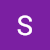`# This Python 3 environment comes with many helpful analytics libraries installed# It is defined by the kaggle/python Docker image: https://github.com/kaggle/docker-python# For example, here's several helpful packages to loadimport numpy as np # linear algebraimport pandas as pd # data processing, CSV file I/O (e.g. pd.read_csv)import matplotlib.pyplot as pltimport osfor dirname, _, filenames in os.walk('/kaggle/input'):    for filename in filenames:        os.path.join(dirname, filename)`
1. Selecting relevant features
2. Handling missing values
3. Data type conversion
4. Data normalization (if necessary)
`train_df = pd.read_csv("/kaggle/input/titanic/train.csv")test_df = pd.read_csv("/kaggle/input/titanic/test.csv")train_df.head()`

## Context Information Provided

`# Removing Irrelevant attributesirrelv_attrs = ['PassengerId','Name','Ticket','Fare']relv_attrs=[x for x in train_df.columns.values if x not in irrelv_attrs]train_df_tmp=train_df[relv_attrs]# Removing Irrelevant attributes from test sestrelv_attrs=[x for x in test_df.columns.values if x not in irrelv_attrs]test_df_tmp=test_df[relv_attrs]`

## Handling Missing Values

`pd.concat([train_df_tmp,test_df_tmp]).isnull().apply(lambda x: x.any(), axis=0)Survived     TruePclass      FalseSex         FalseAge          TrueSibSp       FalseParch       FalseCabin        TrueEmbarked     Truedtype: bool`

## Age

`grouped_train_df=train_df[['Survived','Sex','Age','Pclass']].groupby(['Pclass','Survived','Sex'])['Age'].mean()grouped_train_df.unstack().unstack().plot(kind='bar', figsize=(15,8),                                          title="Fig 1: Age vs Pclass based upon gender & survived",                                         xlabel="Passenger class",                                         ylabel="Average age",                                         colormap='nipy_spectral')<AxesSubplot:title={'center':'Fig 1: Age vs Pclass based upon gender & survived'}, xlabel='Passenger class', ylabel='Average age'>`
`# Filling out missing values for Agecalc_NA_vals=train_df_tmp[['Sex','Age','Pclass']].groupby(['Pclass','Sex'])['Age'].mean().reset_index().rename(columns={"Age":"Missing_Age"})print("Values used to replace missing age")print(calc_NA_vals)train_df_tmp=train_df_tmp.merge(calc_NA_vals, how='left',on=['Sex','Pclass'])test_df_tmp=test_df_tmp.merge(calc_NA_vals, how='left',on=['Sex','Pclass'])train_df_tmp['Age'].fillna(train_df_tmp['Missing_Age'], inplace=True)test_df_tmp['Age'].fillna(test_df_tmp['Missing_Age'], inplace=True)Values used to replace missing age   Pclass     Sex  Missing_Age0       1  female    34.6117651       1    male    41.2813862       2  female    28.7229733       2    male    30.7407074       3  female    21.7500005       3    male    26.507589`

## Embarked

`grouped_emb_train_df=train_df[['PassengerId','Embarked']].groupby(['Embarked'])['PassengerId'].count()grouped_emb_train_df.plot(kind='bar', figsize=(15,8),                          title="Fig 2: Count vs Embarked",                         xlabel="Embarked location",                         ylabel="Total count",                         colormap='seismic')<AxesSubplot:title={'center':'Fig 2: Count vs Embarked'}, xlabel='Embarked location', ylabel='Total count'>`
`# Filling the NA values for Embarkedtrain_df_tmp['Embarked'].fillna('S', inplace=True)test_df_tmp['Embarked'].fillna('S', inplace=True)`

## Cabin

`# Fill all NA by others and count valuestrain_df['Cabin'].fillna('Others').value_counts()Others         687B96 B98          4C23 C25 C27      4G6               4F33              3              ... E77              1D9               1B94              1A7               1C70              1Name: Cabin, Length: 148, dtype: int64`
`df['Cabin_Type'] = # First letter from the Cabindf['Cabin_Count']=# Number of Cabins# These are the unique first letter for the data points that have cabin# I would use a different letter to fill the Null values, i.e. Z(pd.concat([train_df,test_df]))[~(pd.concat([train_df,test_df]))["Cabin"].isnull()]['Cabin'].str.unique()array(['C', 'E', 'G', 'D', 'A', 'B', 'F', 'T'], dtype=object)# Transforming Cabin to Two separate attributestrain_df_tmp_ = train_df_tmp[~train_df_tmp["Cabin"].isnull()][["Cabin"]]train_df_tmp_["Cabin_fl"] = train_df_tmp_["Cabin"].str # Get first lettertrain_df_tmp_["Cabin_cnt"]= train_df_tmp_["Cabin"].str.strip().str.split(' ').apply(lambda x: len(x)) # Get cabin counttrain_df_tmp=train_df_tmp.join(train_df_tmp_[["Cabin_fl","Cabin_cnt"]],  lsuffix='_left', rsuffix='_right') # merge back to original dataframe# Same process with test dataframetest_df_tmp_ = test_df_tmp[~test_df_tmp["Cabin"].isnull()][["Cabin"]]test_df_tmp_["Cabin_fl"] = test_df_tmp_["Cabin"].str # Get first lettertest_df_tmp_["Cabin_cnt"]= test_df_tmp_["Cabin"].str.strip().str.split(' ').apply(lambda x: len(x)) # Get cabin counttest_df_tmp=test_df_tmp.join(test_df_tmp_[["Cabin_fl","Cabin_cnt"]], lsuffix='_left', rsuffix='_right') # merge back to original dataframe`
`train_df_tmp['Cabin_cnt'].fillna(0, inplace=True)train_df_tmp['Cabin_fl'].fillna('Z', inplace=True)test_df_tmp['Cabin_cnt'].fillna(0, inplace=True)test_df_tmp['Cabin_fl'].fillna('Z', inplace=True)train_df_tmp.head()`

## Data Type Conversion

`# Sex Categorial to Numericaltrain_df_tmp['Sex'].replace({'male':0,'female':1}, inplace=True)train_df_tmp=train_df_tmp.join(pd.get_dummies(train_df_tmp['Pclass'], prefix='Pclass'))train_df_tmp=train_df_tmp.join(pd.get_dummies(train_df_tmp['Embarked'], prefix='Embarked'))train_df_tmp=train_df_tmp.join(pd.get_dummies(train_df_tmp['Cabin_fl'], prefix='Cabin_fl'))# For test dataframetest_df_tmp['Sex'].replace({'male':0,'female':1}, inplace=True)test_df_tmp=test_df_tmp.join(pd.get_dummies(test_df_tmp['Pclass'], prefix='Pclass'))test_df_tmp=test_df_tmp.join(pd.get_dummies(test_df_tmp['Embarked'], prefix='Embarked'))test_df_tmp=test_df_tmp.join(pd.get_dummies(test_df_tmp['Cabin_fl'], prefix='Cabin_fl'))`

## Embedded Method

`from sklearn.linear_model import LassoCVmodel = LassoCV(random_state=0)# Only numerical attributes are selected to fit into the modelcols_req=['Sex', 'Age', 'SibSp', 'Parch', 'Cabin_cnt', 'Pclass_1','Pclass_2', 'Pclass_3', 'Cabin_fl_A', 'Cabin_fl_B', 'Cabin_fl_C','Cabin_fl_D', 'Cabin_fl_E', 'Cabin_fl_F', 'Cabin_fl_G','Cabin_fl_T', 'Cabin_fl_Z','Embarked_C','Embarked_Q','Embarked_S']model.fit(train_df_tmp[cols_req],train_df_tmp.Survived)LassoCV(random_state=0)coef = pd.Series(model.coef_, index = train_df_tmp[cols_req].columns)imp_coef = coef.sort_values()# filtering out all the attributes that have 0 coefficientimp_coef[abs(imp_coef)>0].plot(kind = "barh", title="Fig 3: ", figsize=(15,8))<AxesSubplot:title={'center':'Fig 3: '}>`

## Correlation Method

`cols_req=['Survived','Sex', 'Age', 'SibSp', 'Parch', 'Cabin_cnt', 'Pclass_1','Pclass_2', 'Pclass_3', 'Cabin_fl_A', 'Cabin_fl_B', 'Cabin_fl_C','Cabin_fl_D', 'Cabin_fl_E', 'Cabin_fl_F', 'Cabin_fl_G','Cabin_fl_T', 'Cabin_fl_Z','Embarked_C','Embarked_Q','Embarked_S']cor = train_df_tmp[cols_req].corr()fig, ax = plt.subplots(figsize=(18, 12))im = ax.imshow(cor, cmap='Wistia')ax.set_title("Fig 4: Correlation among the required variables")ax.set_xticks(np.arange(len(cols_req)))ax.set_yticks(np.arange(len(cols_req)))ax.set_xticklabels(cols_req)ax.set_yticklabels(cols_req)plt.setp(ax.get_xticklabels(), rotation=45, ha="right",         rotation_mode="anchor")for i in range(len(cols_req)):    for j in range(len(cols_req)):        text = ax.text(j, i, round(cor.iloc[i, j],2),                       ha="center", va="center", color="w")fig.tight_layout()plt.show()`
`from sklearn.svm import SVCfrom sklearn.pipeline import make_pipelinefrom sklearn.preprocessing import StandardScalerfrom sklearn.model_selection import train_test_splitfrom sklearn.metrics import plot_confusion_matrixfrom sklearn.model_selection import cross_val_scorefrom sklearn.metrics import precision_score, recall_score, f1_score, accuracy_score# All used featuresfeatures=['Sex','Age','Parch','SibSp','Pclass_1','Pclass_3', 'Embarked_C','Embarked_Q','Embarked_S']# Populate test set for the missing columnsfor x in features:    if x not in test_df_tmp.columns.values:        test_df_tmp[x]=0X_train,y_train=train_df_tmp[features].to_numpy(),train_df_tmp["Survived"]clf = make_pipeline(StandardScaler(), SVC(gamma='auto'))clf.fit(X_train, y_train)Pipeline(steps=[('standardscaler', StandardScaler()),                ('svc', SVC(gamma='auto'))])`

## Cross-Validation Scores

`scores = cross_val_score(clf, X_train, y_train, cv=10)scoresarray([0.83333333, 0.83146067, 0.7752809 , 0.87640449, 0.86516854,       0.82022472, 0.83146067, 0.76404494, 0.86516854, 0.86516854])`

## Confusion Matrix & Metrics

`X_train, X_test, y_train, y_test = train_test_split(X_train, y_train, random_state=0)clf.fit(X_train, y_train)plot_confusion_matrix(clf, X_test, y_test)<sklearn.metrics._plot.confusion_matrix.ConfusionMatrixDisplay at 0x7f6f9bba7370>`
`func_vars = [('precision_score',precision_score),('recall_score',recall_score),('f1_score',f1_score),('accuracy_score',accuracy_score)]y_pred = clf.predict(X_test)for lab,fn in func_vars:    print(f"The {lab} score for classifier is {round(fn(y_test,y_pred),4)}")The precision_score score for classifier is 0.8143The recall_score score for classifier is 0.6786The f1_score score for classifier is 0.7403The accuracy_score score for classifier is 0.8206`

--

--

--

## More from Dudhraj Sandeep

Just a data science enthusiast.

Love podcasts or audiobooks? Learn on the go with our new app.

## How Programming Pathshala got started?## Beginner’s Guide to Setting up NGINX on Ubuntu 18.04## What it’s like to be a Software Engineer## Interacting with webcam and video file and some drawing with OPEN CV — PART-II## CS373 Spring 2021: Week 2## Aligning business and IT: 3 tips for innovative software development## Dudhraj Sandeep

Just a data science enthusiast.

## Used Car Price prediction & analysis## Unsupervised Machine Learning: What, Why and How..? Example, Using Python## Predicting HDB Resale Prices in Singapore during COVID-19## How to Deal with Categorical Features for Machine Learning